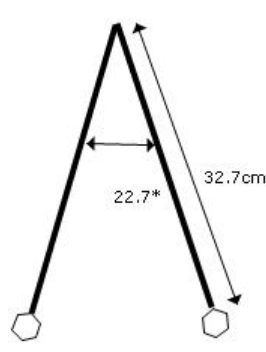In: Physics

# How far does the pendulum bob (the ball at the end of the rope) travel in one complete cycle of motion if its length is 32.7 cm?A pendulum (shown in the figure) sweeps out an angle of 22.7° during its motion. How far does the pendulum bob (the ball at the end of the rope) travel in one complete cycle of motion if its length is 32.7 cm?

## Solutions

##### Expert Solution

Concepts and reason

The concepts required to solve this problem are the motion of the pendulum bob and arc length. First, obtain the expression for the distance covered by the pendulum bob (the ball at the end of the rope) when it travels in one complete cycle of motion using the expression for the arc length. Finally, calculate the distance covered by the pendulum bob (the ball at the end of the rope) when it travels in one complete cycle of motion by using the values of the angle made by the pendulum bob and the length of the pendulum.

Fundamentals

The expression for the arc length when the pendulum bob travels back and forth along an arc during its cycle is, $$\ell=R \theta$$

Here, $$R$$ is the radius of the circular path of the pendulum bob, and $$\boldsymbol{\theta}$$ is the angle made by the angle subtended to the arc.

The expression for the arc length when the pendulum bob travels back and forth along an arc during its cycle is,

$$\ell=R \theta$$

So, the total distance covered by the pendulum bob in one cycle along its path when it needs to go back and forth and for a full cycle is, $$d=2 \ell$$

Substitute $$R \boldsymbol{\theta}$$ for $$\ell$$.

$$d=2 R \theta$$

The arc length when the pendulum bob travels back and forth along an arc during its cycle is depending on the radius of the circular path of the bob and the angle made by the angle subtended to the arc.

The total distance covered by the pendulum bob in one cycle along its path is, $$d=2 R \theta$$

Substitute $$32.7 \mathrm{~cm}$$ for $$R$$ and $$22.7^{\circ}$$ for $$\theta$$. $$d=2(32.7 \mathrm{~cm})\left(22.7^{\circ}\right)\left(\frac{\pi \mathrm{rad}}{180^{\circ}}\right)$$

$$=25.9 \mathrm{~cm}$$

The total distance covered by the pendulum bob in one cycle along its path is depending on the arc length angle made by the pendulum bob. The total distance covered by the pendulum bob in one cycle along its path is two times the arc length.

The total distance covered by the pendulum bob in one cycle along its path is $$25.9 \mathrm{~cm}$$.